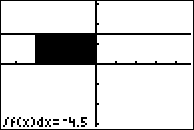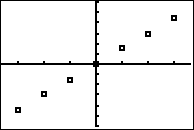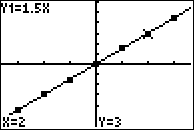# Activities

••• ##### Subject Area

• Math: Calculus: Antiderivatives and Slope Fields

• ##### Author9-12

50 Minutes

• ##### Device
• TI-83 Plus Family
• TI-84 Plus
• TI-84 Plus Silver Edition
•TI-84 Plus C Silver Edition
•TI-84 Plus CE
• ##### Other Materials
This is Activity 16 from the EXPLORATIONS Book:
Calculus Activities.

## Accumulation Functions#### Activity Overview

In this activity, students examine functions defined by a definite integral. They create tables and graphs for integration functions. They understand the foundation of the Fundamental Theorem of Calculus.

#### Key Steps

•Students will create tables and graphs for functions of in the form of a definite integral.

•Students will use the graphing handheld to produce a scatter plot.

•Students will understand the foundation of the Fundamental Theorem of Calculus.